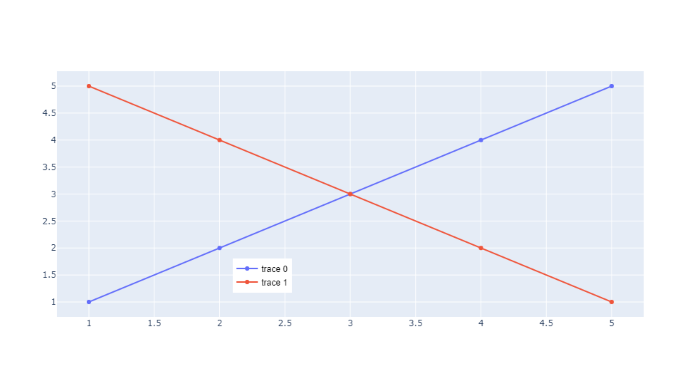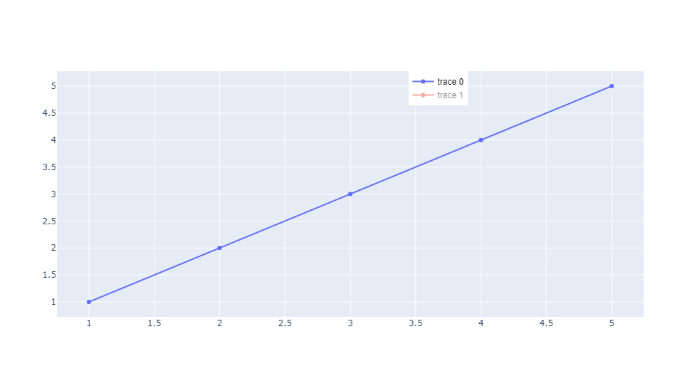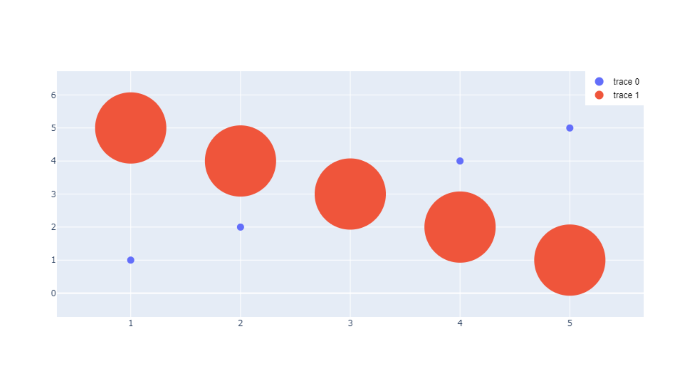Open in App
Not now

# How to position legends inside a plot in Plotly-Python?

• Last Updated : 28 Nov, 2021

In this article, we will learn How to hide legend with Plotly Express and Plotly. A legend is an area describing the elements of the graph. In the plotly legend is used to Place a legend on the axes.

Example 1:

In this example, we are positioning legends inside a plot with the help of method fig.update_layout(), by passing the position as x=0.3 and y=0.1.

## Python3

 `# importing packages``import` `plotly.graph_objects as go`` ` `# using medals_wide dataset``fig ``=` `go.Figure()`` ` `# plotting the scatter chart``fig.add_trace(go.Scatter(``    ``x``=``[``1``, ``2``, ``3``, ``4``, ``5``],``    ``y``=``[``1``, ``2``, ``3``, ``4``, ``5``],``))`` ` `# plotting the scatter chart``fig.add_trace(go.Scatter(``    ``x``=``[``1``, ``2``, ``3``, ``4``, ``5``],``    ``y``=``[``5``, ``4``, ``3``, ``2``, ``1``],``))`` ` `#  position legends inside a plot``fig.update_layout(``    ``legend``=``dict``(``        ``x``=``0.3``,  ``# value must be between 0 to 1.``        ``y``=``.``1``,   ``# value must be between 0 to 1.``        ``traceorder``=``"normal"``,``        ``font``=``dict``(``            ``family``=``"sans-serif"``,``            ``size``=``12``,``            ``color``=``"black"``        ``),``    ``)``)`` ` `fig.show()`

Output:Example 2:

In this example, we are positioning legends inside a plot with the help of method fig.update_layout(), by passing the position as x=0.6 and y=1.

## Python3

 `# importing packages``import` `plotly.graph_objects as go`` ` `# using medals_wide dataset``fig ``=` `go.Figure()`` ` `# plotting the scatter chart``fig.add_trace(go.Scatter(``    ``x``=``[``1``, ``2``, ``3``, ``4``, ``5``],``    ``y``=``[``1``, ``2``, ``3``, ``4``, ``5``],``))`` ` `# plotting the scatter chart``fig.add_trace(go.Scatter(``    ``x``=``[``1``, ``2``, ``3``, ``4``, ``5``],``    ``y``=``[``5``, ``4``, ``3``, ``2``, ``1``],``    ``visible``=``'legendonly'``))`` ` `# position legends inside a plot``fig.update_layout(``    ``legend``=``dict``(``        ``x``=``0.6``,  ``# value must be between 0 to 1.``        ``y``=``1``,  ``# value must be between 0 to 1.``        ``traceorder``=``"normal"``,``        ``font``=``dict``(``            ``family``=``"sans-serif"``,``            ``size``=``12``,``            ``color``=``"black"``        ``),``    ``)``)`` ` `fig.show()`

Output:Example 3:

In this example, we are positioning legends inside a plot with the help of method fig.update_layout(), by passing the position as x=0.9 and y=1.

## Python3

 `import` `plotly.graph_objects as go`` ` `fig ``=` `go.Figure()`` ` `fig.add_trace(go.Scatter(``    ``x``=``[``1``, ``2``, ``3``, ``4``, ``5``],``    ``y``=``[``1``, ``2``, ``3``, ``4``, ``5``],``    ``mode``=``'markers'``,``    ``marker``=``{``'size'``: ``10``}``))`` ` `# plotting the scatter chart``fig.add_trace(go.Scatter(``    ``x``=``[``1``, ``2``, ``3``, ``4``, ``5``],``    ``y``=``[``5``, ``4``, ``3``, ``2``, ``1``],``    ``mode``=``'markers'``,``    ``marker``=``{``'size'``: ``100``}``))`` ` `# position legends inside a plot``fig.update_layout(``    ``legend``=``dict``(``        ``x``=``.``9``,  ``# value must be between 0 to 1.``        ``y``=``1``,   ``# value must be between 0 to 1.``        ``traceorder``=``"normal"``,``        ``font``=``dict``(``            ``family``=``"sans-serif"``,``            ``size``=``12``,``            ``color``=``"black"``        ``),``    ``)``)`` ` `fig.update_layout(legend``=``{``'itemsizing'``: ``'constant'``})`` ` `fig.show()`

Output:My Personal Notes arrow_drop_up